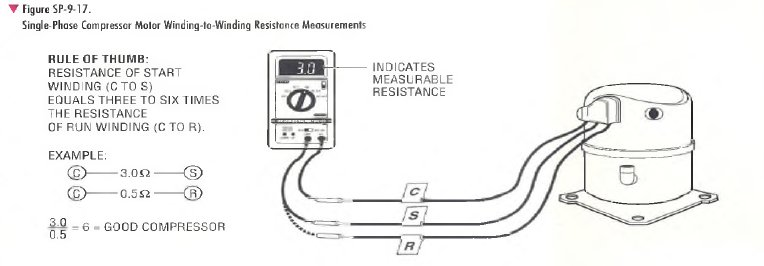﻿ SINGLE-PHASE COMPRESSOR MOTOR WINDING-TO-WINDING RESISTANCE MEASUREMENT PROCEDURE | HVAC Troubleshooting

## SINGLE-PHASE COMPRESSOR MOTOR WINDING-TO-WINDING RESISTANCE MEASUREMENT PROCEDURE

StepExpected Result/Action
13. Check a single-phase compressor motor for winding-to-winding shorts
(partially shorted) windings as follows:
Because of the extremely low resistance values being measured, an accurate
VOM/DMM must be used to perform this step, otherwise a good compressor
may be judged as bad. Set up the VOM/DMM to measure resistance on the
R x 1 ohm scale. Moke sure that the meter is zeroed. Connect the VOM/DMM
across the leads connected to compressor terminals C and S, then measure
and record the resistance of the start winding as shown in Figure SP-9-17.
Connect the VOM/DMM across the leads connected to compressor terminals C
and R, then measure and record the resistance of the run winding.
VOM/DMM indicates a measurable low resistance for both measurements. As a
rule of thumb, the motor windings are usually judged to be good if the resistance
measured across the start winding (C to S) is 3 to 6 times greater than the
resistance measured across the run winding (C to R).
Example 1
Assume that the resistance measured for the start winding is 3.0 ohms and the
run winding is 0.5 ohm. In this example?, the motor windings would be judged to
be good because the start winding is 6 times the resistance o f the run winding
( 3 . 0 + 0 . 5 = 61
Example 2
Assume that the resistance measured for the start winding is 1.25 ohms and the
run winding is 0.5 ohm. In this example> the motor windings would be judged to
be partially shorted because the start winding is only 2.5 times the resistance of
the run winding (1.25 + 0.5 = 2.5). (Start winding resistance in this example is
less than 3 times greater than the run winding resistance.)
Example 3
Assume that the resistance measured for the start winding is 4.0 ohms and the
run winding is about 0.33 ohm. In this example, the motor windings would be
judged to be partially shorted because the start winding is about J 2 times the
resistance of the run winding ( 4 .0 + 0 .3 3 = 12). (Start winding resistance in this
example is more than 6 times greater than the run winding resistance.)